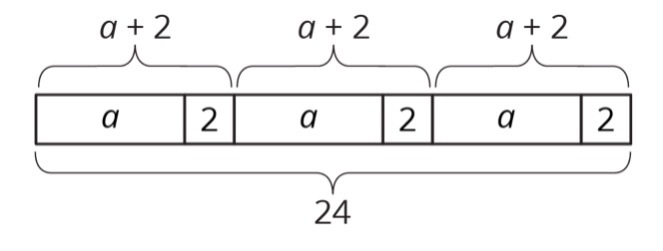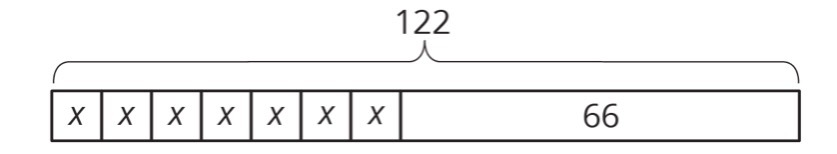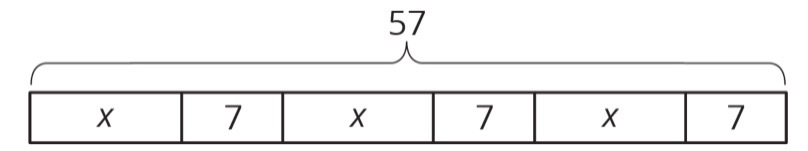PSABM6PB - Student Facing Task---Acc7.3 Lesson 11 Using Equations to Solve Problems (7.EE.B.3, 7.EE.B.4, 7.EE.B.4.a)
Part A)Write a story that could be represented by this tape diagram.

Part B)

Write an equation that could be represented by this tape diagram.

24 = ________

Part A)

Tyler is making invitations to the fair. He has already made some of the invitations, and he wants to finish the rest of them within a week. He is trying to spread out the remaining work, to make the same number of invitations each day. Tyler draws a diagram to represent the situation.How many total invitations is Tyler trying to make?

Type your answer below as a number (example: 5, 3.1, 4 1/2, or 3/2):
Part B)

Type your answer below as a number (example: 5, 3.1, 4 1/2, or 3/2):
Part C)

How many days does he have to finish the invitations?

Type your answer below as a number (example: 5, 3.1, 4 1/2, or 3/2):
Part D)

Explain how each part of the situation is represented in Tyler’s diagram.

Part E)

How many invitations should Tyler make each day to finish his goal within a week?

Type your answer below as a number (example: 5, 3.1, 4 1/2, or 3/2):
Part F)

Part G)

Use Tyler’s diagram to write an equation that represents the situation.

122 = _____________

Part H)

Explain how each part of the situation is represented in your equation.

Part I)

Show how to solve your equation.

Part A)

Noah and his sister are making prize bags for a game at the fair. Noah is putting 7 pencil erasers in each bag. His sister is putting in some number of stickers. After filling 3 of the bags, they have used a total of 57 items.Explain how the diagram represents the situation.

Part B)

Noah writes the equation 3(x+7)=57 to represent the situation. Do you agree with him?

Select one:
Part C)

Part D)

How many stickers is Noah's sister putting in each prize bag?

Type your answer below as a number (example: 5, 3.1, 4 1/2, or 3/2):
Part E)

Part A)

A family of 6 is going to the fair. They have a coupon for $1.50 off each ticket. If they pay$46.50 for all their tickets, how much does a ticket cost without the coupon?

Do not include units ($) in your answer. copied for free from openupresources.org Type your answer below as a number (example: 5, 3.1, 4 1/2, or 3/2): Part B) Explain or show your reasoning. Type your answer below: Part A) Priya, Han, and Elena, are members of the running club at school. Priya was busy studying this week and ran 7 fewer miles than last week. She ran 9 times as far as Elena ran this week. Elena only had time to run 4 miles this week. How many miles did Priya run last week? Do not include units (miles) in your answer. copied for free from openupresources.org Type your answer below as a number (example: 5, 3.1, 4 1/2, or 3/2): Part B) Elena wrote the equation 1/9(x7)=4 to describe the situation. She solved the equation by multiplying each side by 9 and then adding 7 to each side. How does her solution compare to the way you found Priya's miles? Type your answer below: Part C) One day last week, 6 teachers joined 5/7 of the members of the running club in an after-school run. Priya counted a total of 31 people running that day. How many members does the running club have? Do not include units (members) in your answer. Type your answer below as a number (example: 5, 3.1, 4 1/2, or 3/2): Part D) Priya and Han plan a fundraiser for the running club. They begin with a balance of -80 because of expenses. In the first hour of the fundraiser they collect equal donations from 9 parents, which brings their balance to -44. How much did each parent give? Do not include units ($) in your answer.

Type your answer below as a number (example: 5, 3.1, 4 1/2, or 3/2):
Part E)

The running club uses the money they raised to pay for a trip to a canyon. At one point during a run in the canyon, the students are at an elevation of 128 feet. After descending at a rate of 50 feet per minute, they reach an elevation of -472 feet. How long did the descent take?

A musician performed at three local fairs. At the first he doubled his money and spent $30. At the second he tripled his money and spent$54. At the third, he quadrupled his money and spent $72. In the end he had$48 left. How much did he have before performing at the fairs?